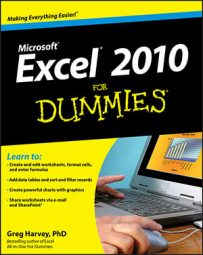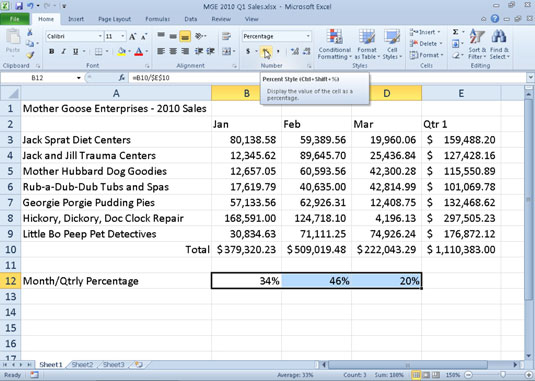##### Excel 2010 For DummiesMany Excel 2010 worksheets use percentages in the form of interest rates, growth rates, inflation rates, and so on. When you apply the Percent Style format to a value that has already been entered in a cell, Excel multiplies the value by 100 and displays the result with a percent sign.

To insert a percentage in a cell as you are entering a value, type the percent sign (%) after the number. To indicate an interest rate of 12 percent, for example, you enter 12% in the cell. When you use this method, Excel assigns a Percent Style number format, divides the value by 100 (that’s what makes it a percentage), and places the result in the cell (0.12 in this example).

Not all percentages in a worksheet are entered by hand in this manner. Some may be calculated by a formula and returned to their cells as raw decimal values. In such cases, you should add a Percent format to convert the calculated decimal values to percentages.Monthly-to-quarterly sales percentages with Percent Style number formatting.

Follow these steps to format numbers in Excel 2010 with the Percent style:

1. Select the cells containing the numbers you want to format.

2. On the Home tab, click the Number dialog box launcher in the bottom-right corner of the Number group.

The Format Cells dialog box appears, with the Number tab on top.

3. In the Category list, select Percentage.

4. Specify the number of decimal places.

Type the number in the Decimal places text box or use the spin arrows to select a number.

5. Click OK.

Click the Percent Style button in the Number group of the Home tab to quickly format the cell selection by converting values to percentages.